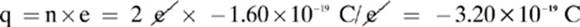﻿ ﻿Electric Fields - Electric Charges, Forces, and Fields - Homework Helpers: Physics

## 5 Electric Charges, Forces, and Fields

### Lesson 5–4: Electric Fields

How can an object exert a force on another object when they aren”t even touching? What is the nature of a force at a distance? The idea of an object interacting on a distant object is easier to visualize when we employ the model of force fields. In this model, you imagine each object surrounded by a field, or area. In the case of gravitational fields, the more massive the object is, the stronger the gravitational field it is surrounded by. In the case of electric fields, objects with greater charges (q) are surrounded by stronger electric fields.

Not all electric fields are uniform in terms of strength. Just as the force of attraction to Earth due to gravity is greatest near its surface, the electrostatic force of attraction or repulsion for an ion is greater as you get closer to it. The electric field strength (E) at a given point in the field is measured in terms of how much force a charged object within it experiences at that position, per units of its charge. Electric field strength is also called electric field intensity.Figure 5.6orThe units used for measuring electric field intensity are newtons/coulomb.

Example 1

Calculate the magnitude of the force that a proton will experience if it is placed at a point in an electric field where the intensity is 0.15 N/C. (Recall that the charge on one proton is 1.60 × 10–19 C.)We treat tiny charged objects as point charges, meaning we treat them as if they take up no space and a uniform radial field surrounds them. Sometimes you won”t be given the charge of a test charge or the force experienced by the test charge. In these situations you can combine the formula for electric field intensity with Coulomb”s law to find the strength of the electric field generated by a point charge at a given distance.Example 2

Determine the strength of the electric field at a distance of 3.0 × 10–3 m from an oxide (O2–) ion.

Convert: Remember: An oxide (O2–) ion has two extra electrons, so we find its net charge by multiplying the elementary charge (e) by 2.Because we are finding only the magnitude (strength) of the electric field, and not the direction, we can use the absolute value of the charge.By measuring the strength of an electric field at many different spots, you can “map” it out in the same way that you can make a topographic map from many measurements of altitude. An electric field diagram is a qualitative representation of such a map, following these conventions:

1. Field lines are drawn as arrows, pointing in the direction of the force a positive test charge would experience at that point in the field. (For curved lines, the force experienced at that point is tangent to the curve.)

2. Field lines are never drawn crossing each other.

3. The more lines per given unit of area, the stronger the field at that point.Figure 5.7

Not all charged objects are treated as point charges. A capacitor, which is a device that can be used to store charge, is made up of two metallic plates. When these plates are charged, one will have a net positive charge and the other will have a net negative charge. The type of field between the plates is called a uniform electric field.

In the type of electric field formed between two charged plates, the electric potential (V) decreases linearly with the distance, not with the square of the distance, as in the field surrounding a point charge. The formula for calculating the average strength of a uniform electric field is:Figure 5.8where d is the distance between the plates, and V is the voltage, or potential difference between the plates.

Lesson 5–4 Review

1. How would the force on an electron in an electric field compare to the force experienced by a proton in the same location in the same electric field?

2. If an electron experiences an electrostatic force of –1.1 × 10–18 N in a field, calculate the electric field strength.

3. Calculate the magnitude of the electric field strength at a distance of 1.5 × 10–4 m from a proton.

4. Calculate the magnitude of the electric force experienced by a helium (He2+) ion at a point in an electric field where the field intensity is 2.2 N/C.

﻿